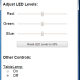# Driving Relays with the ESP8266

The ESP8266 has a current limit of 12ma, so we have to take care when driving relays.

First I start with a 817C Optoisolator. The datasheet says says the IR LED drops 1.2v, so I plug 3.3v source voltage, 1.2v forward voltage, and 12ma forward current into the calculator at http://led.linear1.org/led.wiz and I get a 180 ohm resistor as the optimum value.

Now, according to the datasheet for the 817C, the Current transfer ratio at 12ma is around 120%, so I can only conduct around 14-15ma through the photo transistor, not nearly enough to trigger a relay.

Let’s have the photo transistor trigger an additional transistor, one that can allow the full current needed by the coil of the relay. I have chosen a 2N5551, since the datasheet says it can handle 600ma and 5v.

Now the relay. I chose a HK19F 5v 125ohm relay which, according to Ohm’s law and the datasheet, draws 40ma. It’s a DPDT relay (most commercial modules use a SPDT), but the contacts are only rated for 1A or so.

Don’t forget a flyback diode for that relay. I used a 1N4148, which, according to the datasheet, easily handles the current and voltage of the relay.

If you want, you could add a LED / 150 ohm resistor across the coil of the relay as a visual indicator.

Now a simple

digitalWrite(5, HIGH);

makes it all happen!

UPDATE: 3v MOSFET instead of optoisolator. Simpler and cheaper!Subscribe
Notify ofInline FeedbacksJeff Verive
1 year ago

I prefer to design circuits like this starting with the load and working backwards, though the ESP8266’s maximum output current limitations warrant solving from both ends or, at least, keeping the limitations in mind when solving backwards from the load.

Since the relay coil resistance is 125 ohms +/- 10% and the supply voltage is 5V, the relay current for a saturated BJT as a switch [Vce(sat) of about 0.2V] is 4.8V/125Ω, which is 38.4mA. This is close enough to the nominal value of 40mA, so we can use either.

The 2N5551 is a fine choice for this application, and for use as a saturated switch the datasheet gives a current gain (Ic/Ib) of 10, despite the minimum gain of 30 in the active region. This saturated gain of 10 is extremely common for such transistors, so it’s a safe value to assume for such circuits; but make sure to verify this at some point in the design cycle because making changes after the circuit is built is usually harder.

Armed with this knowledge, it’s clear that the base current should be at least 3.84mA (or 4 mA, if you prefer to use Ic of 40mA as discussed above). The base current is equal to (Vb – Vbe)/Rb, and we can look at the datasheets to find that Vb is around 4.8V, and Vbe is around 0.8V (but could be closer to 1V, so to be conservative I would use 1V for calculations. Therefore, for a base current of 4mA, the base resistor calculation gives Rb = (4.8V – 1V)/0.004A = 950Ω. The chosen value of 510 is somewhat low for this application, but it’s not an unreasonable choice. I much prefer to see a litle more base current than too little current. If a worst-case design is performed, I’m certain that 510 ohms is a better choice than my nominal calculation of 950Ω!

This is a good time to revisit the ESP8266’s maximum output current limitation of 12mA, because this affects our choice of opto-coupler. Specifically, the opto-coupler must be able to give an output of at least 4mA for an input current of 12mA – so the CTR (current transfer ratio) must be at least 4mA/12mA * 100% = 33.3%. The opto-coupler chosen here meets this requirement very easily, so we can use it without concerns.

Finally, it’s instructive to look at the calculation for the opto-coupler’s input resistance. We only need 4mA from the opto-coupler, and the CTR of a bit over 100% at 4mA output tells us that the input current needs to be at least 4mA BUT no more than 12mA. At 4mA, the ESP8266’s output high voltage will be around 3.1V, and with the opto-coupler’s input voltage (Vf) of around 1.1V, the maximum resistance is around 475 ohms. To get 12mA the ESP8266’s output voltage is around 2.6V and Vf is around 1.2V, so the resistance calculation gives a minimum of 121Ω. Brian’s choice of 180Ω fits well in this range, so overall I have to give Brian a hand. As you can see, there’s often a lot of work required even in relatively simple designs. If you don’t want to have to work out all of the calculations, a good circuit simulator can be very useful. Just understand that the simulation results are only as good as the device models, so it’s helpful to do the calculations so that you develop enough intuition to help you decide when to question the simulators results!5 years ago

This only makes sens if you need electrical isolation (i.e. a separate power source to drive the relay), if not just go without the optoisolator. If yes, i'd prefer a solid state relay

2
0